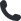Need help? Call us on0800 970 0077

Monday to friday from 9am to 5:30pm

# Convert gas units into kWhThe article below explains how to convert gas units into kWh, which will help to ensure your supplier is billing you correctly.

If your energy bills are too high, you can save an average of £491* by switching and we’ll do all the work for you.

## How do I convert gas units to kWh?

First, you need to determine whether you have an imperial meter or a metric meter:

• For imperial meters, your reading will be in hundreds of cubic feet (100ft cubed).
• Metric meter readings are recorded in cubic meters (m cubed).

I have an imperial meter

To convert imperial meter readings to kWh, all you need to do is:

2. Convert from imperial to metric by multiplying the units by 2.83
3. Multiply by volume correction factor (1.02264).
4. Multiply by calorific value (40.0).
5. Divide by kWh conversion factor (3.6).

If your imperial meter measures in cubic feet rather than hundreds of cubic feet, you’ll need to use 0.0283 for step 2 rather than 2.83. If it uses thousands of cubic feet, you’ll need to use 28.3 instead. Your meter will usually say ‘x100’ or ‘x1000’ to indicate the higher units of measurement.

I have a metric gas meter

Calculate your reading as explained in the section above. Convert from imperial to metric by multiplying the units by 2.83. Multiply by volume correction factor (1.02264). Multiply by calorific value (40.0). Divide by kWh conversion factor (3.6). To find out if you’re paying too much for energy, take a look at the average energy bill for your size of house.

## What’s the volume correction factor, when converting gas units to kWh?

The volume correction factor is used to take into account the temperature, pressure and atmospheric conditions at a property. This factor is typically 1.02264 unless your household has unusual atmospheric conditions. You can usually find this number on your gas bill.

## What is a calorific value, when converting gas units to kWh?

A calorific value (CV) is a measurement of the amount of energy contained in gas, measured in megajoules per cubic meter (MJ/m cubed).

The CV of the gas at each Local Distribution Zone is continually measured by the National Grid who sends this figure to your gas supplier, who then uses it for their calculations. Typically, a gas supply has a CV between 37.5 and 43.0 MJ/m cubed. You can use 40.0 as a default number if you don’t have the exact figure.